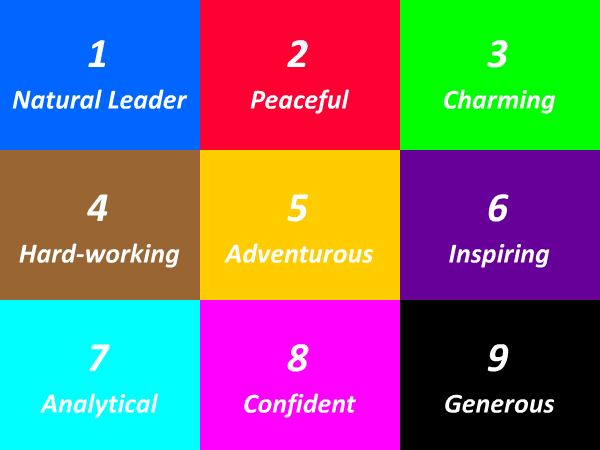# 91 Times Table - Generate Multiplication Table of 91

91 Times Table
1x91=91
2x91=182
3x91=273
4x91=364
5x91=455
6x91=546
7x91=637
8x91=728
9x91=819
10x91=910
11x91=1001
12x91=1092
13x91=1183
14x91=1274
15x91=1365
16x91=1456
17x91=1547
18x91=1638
19x91=1729
20x91=1820
21x91=1911
22x91=2002
23x91=2093
24x91=2184
25x91=2275
91 Times Table
26x91=2366
27x91=2457
28x91=2548
29x91=2639
30x91=2730
31x91=2821
32x91=2912
33x91=3003
34x91=3094
35x91=3185
36x91=3276
37x91=3367
38x91=3458
39x91=3549
40x91=3640
41x91=3731
42x91=3822
43x91=3913
44x91=4004
45x91=4095
46x91=4186
47x91=4277
48x91=4368
49x91=4459
50x91=4550
91 Times Table
51x91=4641
52x91=4732
53x91=4823
54x91=4914
55x91=5005
56x91=5096
57x91=5187
58x91=5278
59x91=5369
60x91=5460
61x91=5551
62x91=5642
63x91=5733
64x91=5824
65x91=5915
66x91=6006
67x91=6097
68x91=6188
69x91=6279
70x91=6370
71x91=6461
72x91=6552
73x91=6643
74x91=6734
75x91=6825

## Test Your Skills Here

The 91 times table quiz is very useful in the improvement of kid's multiplication and maths skills. Here you will find a selection of times table tests designed to help your child to learn and practice their times tables. Fill in your answers. Once you have entered all the answers, click on 'Check' button to see whether you have got them all correct. If your answers is Wrong then we will show you the 'Correct' answer.

Practice Excersise
91 x = 546
91 x 10 =
91 x 30 =
91 x = 2730
91 x 8 =
91 x 16 =
91 x = 819
91 x 23 =
91 x = 1092
91 x = 2457#### More Tables#### Top Search Quesries for this page:

• 91 times table
• 91 times tables
• table of 91
• 91 multiplication table
• multiplication chart 91
• multiplication of 91
• 91 table maths### Spreading Knowledge Across the World

USA - United States of America  Canada  United Kingdom  Australia  New Zealand  South America  Brazil  Portugal  Netherland  South Africa  Ethiopia  Zambia  Singapore  Malaysia  India  China  UAE - Saudi Arabia  Qatar  Oman  Kuwait  Bahrain  Dubai  Israil  England  Scotland  Norway  Ireland  Denmark  France  Spain  Poland  and  many more....